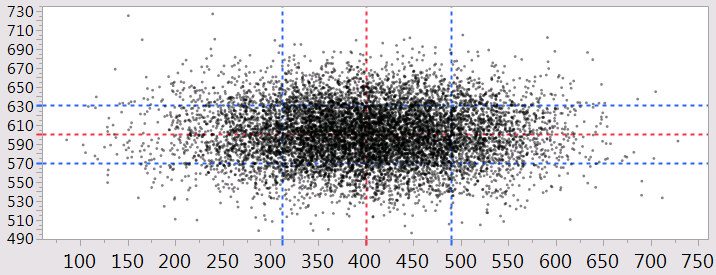Our World Statistics Day conversations have been a great reminder of how much statistics can inform our lives. Do you have an example of how statistics has made a difference in your life? Share your story with the Community!
Choose Language Hide Translation Bar

## Problem

You need to automate adding reference lines to a chart.

## Solution

Use JSL to run a summary on the data. Take the summary values and insert them into the graph builder script.

``````// here's an example table; the x1 and x2 have different means and sigmas
dt = New Table( "Untitled",
New Column( "x1", Numeric, "Continuous", Format( "Best", 12 ), Formula( Random Normal( 400, 90 ) ) ),
New Column( "x2", Numeric, "Continuous", Format( "Best", 12 ), Formula( Random Normal( 600, 30 ) ) )
);
// the summary will be a single row with the requested information
dtsummary = dt << Summary(
Mean( :x1 ),
Mean( :x2 ),
Std Dev( :x1 ),
Std Dev( :x2 ),
Freq( "None" ),
Weight( "None" )
);
// grab the values of interest in simpler variable names
x1Mean = dtsummary:name("Mean(x1)"); // row one has the answers
x1StdDev = dtsummary:name("Std Dev(x1)");
x2Mean = dtsummary:name("Mean(x2)");
x2StdDev = dtsummary:name("Std Dev(x2)");
// make a graph
gb = dt<<Graph Builder( Size( 512, 442 ), Show Control Panel( 0 ),
Variables( X( :x1 ), Y( :x2 ) ), Elements( Points( X, Y, Legend( 5 ) ) ),
// add ref lines. You can get a script like this GraphBuilder script by
// making a graph builder interactively, then using the red triangle->get script.
// below, the numbers in the script have been replaced with the variables above.
SendToReport( Dispatch( {}, "x1", ScaleBox,
{Add Ref Line( x1Mean, "Dotted", "Red", "", 2 ),
Add Ref Line( x1Mean+x1StdDev, "Dotted", "Blue", "", 2 ),
Add Ref Line( x1Mean-x1StdDev, "Dotted", "Blue", "", 2 )
}
),
Dispatch( {}, "x2", ScaleBox,
{Add Ref Line( x2Mean, "Dotted", "Red", "", 2 ),
Add Ref Line( x2Mean+x2StdDev, "Dotted", "Blue", "", 2 ),
Add Ref Line( x2Mean-x2StdDev, "Dotted", "Blue", "", 2 )
}
),
Dispatch( {}, "Graph Builder", FrameBox,
{Marker Size( 1 ), Transparency( 0.5 )} ) )
);
// make the data space square
report(gb)[framebox(1)]<<Size to Isometric;
``````

## Discussion

You can use close(dtsummary,"nosave"); to clean up the extra table. Build the chart interactively the first time, add some dummy reference lines, then save the script. You do not have to write all that JSL yourself!Reference lines automatically added with JSL